Next: Representing the Objective Function Up: Graphical solution of 2-var Previous: The solution space of

#### The solution space of a single inequality constraint

Consider the constraint: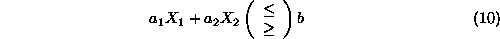The solution space of this constraint is one of the closed half-planes defined by the equation: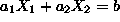. To show this, let us consider a point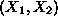which satsifies Equation 10 as equality, and another point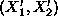for which Equation 10 is also valid. For any such pair of points, it holds that: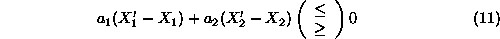Interpreting the left side of Eq. 11 as the inner (dot) product of the two vectors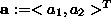and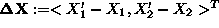, and recognizing that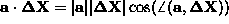, it follows that line, itself, can be defined by pointand the set of pointssuch that vector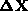is at right angles with vector. Furthermore, the set of pointsthat satisfy the > (<) part of Equation 11 have the vectorforming an acute (obtuse) angle with vector, and therefore, they are ``above'' (``below'') the line. Hence, the set of points satisfying each of the two inequalities implied by Equation 10 is given by one of the two half-planes the boundary of which is defined by the corresponding equality constraint. Figure 1 summarizes the above discussion.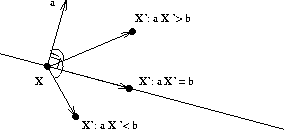Figure 1: Half-planes: the feasible region of a linear inequality

An easy way to determine the half-plane depicting the solution space of a linear inequality, is to draw the line depicting the solution space of the corresponding equality constraint, and then test whether the point (0,0) satisfies the inequality. In case of a positive answer, the solution space is the half-space containing the origin, otherwise, it is the other one.

From the above discussion, it follows that the feasible region for the prototype LP of Equation 5 is the shaded area in the following figure: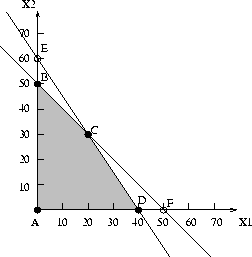Figure 2: The feasible region of the prototype example LPNext: Representing the Objective Function Up: Graphical solution of 2-var Previous: The solution space of

UAL Data
Fri Jun 20 15:03:05 CDT 1997